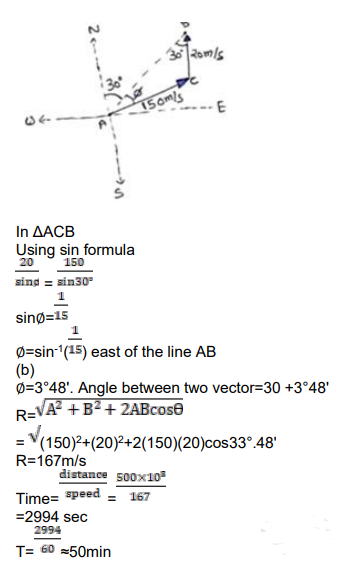# An aero plane has to go from point A

Question:

An aero plane has to go from point $A$ to another point $B, 500 \mathrm{~km}$ away due to $30^{\circ}$ east of north. A wind is blowing due north at a seed of $20 \mathrm{~m} / \mathrm{s}$. The air speed of the plane is $150 \mathrm{~m} / \mathrm{s}$.

(a) Find the direction in which the pilot should head the plane to reach the point $B$.

(b) Find the time taken by the plane to gr from $A$ to $B$.

Solution:

(a)